# 8.6 Buffers

Learning Objectives

By the end of this section, you will be able to:

• Describe the composition and function of acid–base buffers
• Calculate the pH of a buffer before and after the addition of added acid or base

A solution containing appreciable amounts of a weak conjugate acid-base pair is called a buffer solution, or a buffer. Buffer solutions resist a change in pH when small amounts of a strong acid or a strong base are added (Figure 8.6.1). A solution of acetic acid and sodium acetate (CH3COOH + CH3COONa) is an example of a buffer that consists of a weak acid and its salt. An example of a buffer that consists of a weak base and its salt is a solution of ammonia and ammonium chloride (NH3(aq) + NH4Cl(aq)).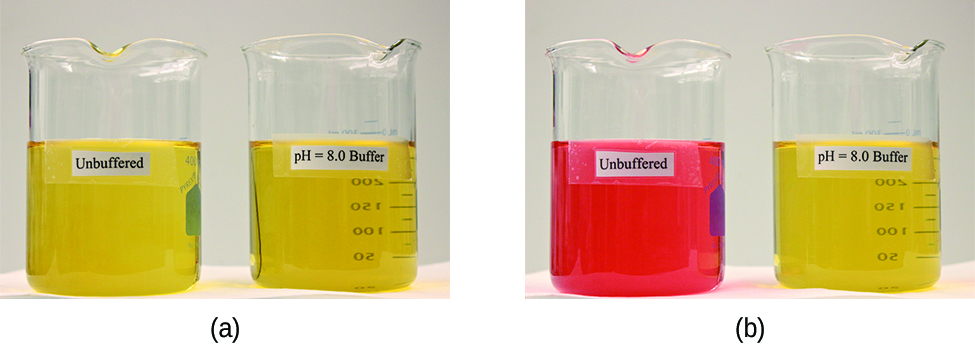Figure 8.6.1 – (a) The buffered solution on the left and the unbuffered solution on the right have the same pH (pH 8); they are basic, showing the yellow color of the indicator methyl orange at this pH. (b) After the addition of 1 mL of a 0.01-M HCl solution, the buffered solution has not detectably changed its pH but the unbuffered solution has become acidic, as indicated by the change in color of the methyl orange, which turns red at a pH of about 4. (credit: modification of work by Mark Ott)

## How Buffers Work

To illustrate the function of a buffer solution, consider a mixture of roughly equal amounts of acetic acid and sodium acetate. The presence of a weak conjugate acid-base pair in the solution imparts the ability to neutralize modest amounts of added strong acid or base. For example, adding strong base to this solution will neutralize hydronium ion and shift the acetic acid ionization equilibrium to the right, partially restoring the decreased H3O+ concentration: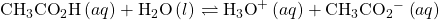Likewise, adding strong acid to this buffer solution will neutralize acetate ion, shifting the above ionization equilibrium right and returning [H3O+] to near its original value. (Figure 8.6.2) provides a graphical illustration of the changes in conjugate-partner concentration that occur in this buffer solution when strong acid and base are added. The buffering action of the solution is essentially a result of the added strong acid and base being converted to the weak acid and base that make up the buffer’s conjugate pair. The weaker acid and base undergo only slight ionization, as compared with the complete ionization of the strong acid and base, and the solution pH, therefore, changes much less drastically than it would in an unbuffered solution.

Activity 8.6.1 – pH Changes in Buffered and Unbuffered Solutions

Acetate buffers are used in biochemical studies of enzymes and other chemical components of cells to prevent pH changes that might affect the biochemical activity of these compounds.

(a) Calculate the pH of an acetate buffer that is a mixture with 0.10 M acetic acid and 0.10 M sodium acetate.

(b) Calculate the pH after 1.0 mL of 0.10 NaOH is added to 100 mL of this buffer.

(c) For comparison, calculate the pH after 1.0 mL of 0.10 M NaOH is added to 100 mL of a solution of an unbuffered solution with a pH of 4.74.

## Solution

(a) Following the ICE approach to this equilibrium calculation yields the following: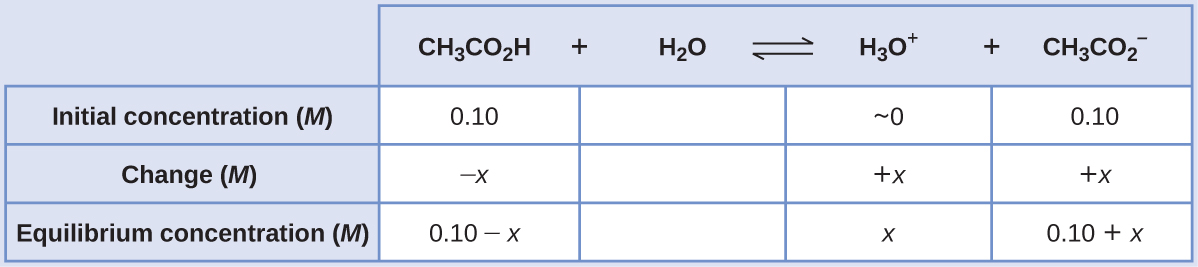Substituting the equilibrium concentration terms into the Ka expression, assuming x << 0.10, and solving the simplified equation for x yields: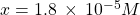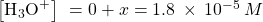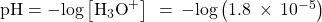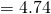(b) Calculate the pH after 1.0 mL of 0.10 M NaOH is added to 100 mL of this buffer.

Adding strong acid will neutralize some of the acetic acid, yielding the conjugate base acetate ion. Compute the new concentrations of these two buffer components, then repeat the equilibrium calculation of part (a) using these new concentrations: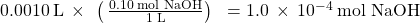The initial molar amount of acetic acid is: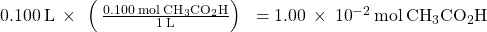The amount of acetic acid remaining after some is neutralized by the added base is: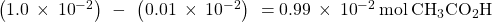The newly formed acetate ion, along with the initially present acetate, gives a final acetate concentration of: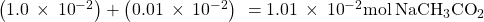Compute molar concentrations for the two buffer components: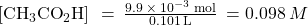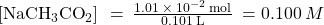Using these concentrations, the pH of the solution may be computed as in part (a) above, yielding pH = 4.75 (only slightly different from that prior to adding the strong base).

(c) For comparison, calculate the pH after 1.0 mL of 0.10 M NaOH is added to 100 mL of a solution of an unbuffered solution with a pH of 4.74.

The amount of hydrogen ion initially present in the solution is: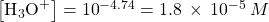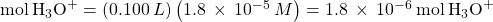The amount of hydroxide ion added to the solution is: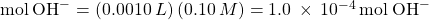The added hydroxide will neutralize hydronium ion via the reaction: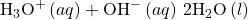The 1:1 stoichiometry of this reaction shows that an excess of hydroxide has been added (greater molar amount than the initially present hydronium ion).

The amount of hydroxide ion remaining is: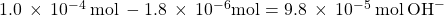corresponding to a hydroxide molarity of: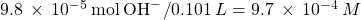The pH of the solution is then calculated to be:In this unbuffered solution, addition of the base results in a significant rise in pH (from 4.74 to 10.99) compared with the very slight increase observed for the buffer solution in part (b) (from 4.74 to 4.75).

Show that adding 1.0 mL of 0.10 M HCl changes the pH of 100 mL of a 1.8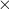10−5M HCl solution from 4.74 to 3.00.

Initial pH of 1.810−5M HCl; pH = −log[H3O+] = −log[1.810−5] = 4.74
Moles of H3O+ in 100 mL 1.810−5M HCl; 1.810−5 moles/L0.100 L = 1.810−6
Moles of H3O+ added by addition of 1.0 mL of 0.10 M HCl: 0.10 moles/L0.0010 L = 1.010−4 moles; final pH after addition of 1.0 mL of 0.10 M HCl: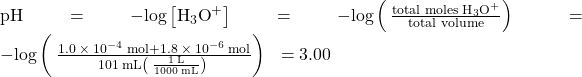## Buffer Capacity

Buffer solutions do not have an unlimited capacity to keep the pH relatively constant (Figure 8.6.3). Instead, the ability of a buffer solution to resist changes in pH relies on the presence of appreciable amounts of its conjugate weak acid-base pair. When enough strong acid or base is added to substantially lower the concentration of either member of the buffer pair, the buffering action within the solution is compromised.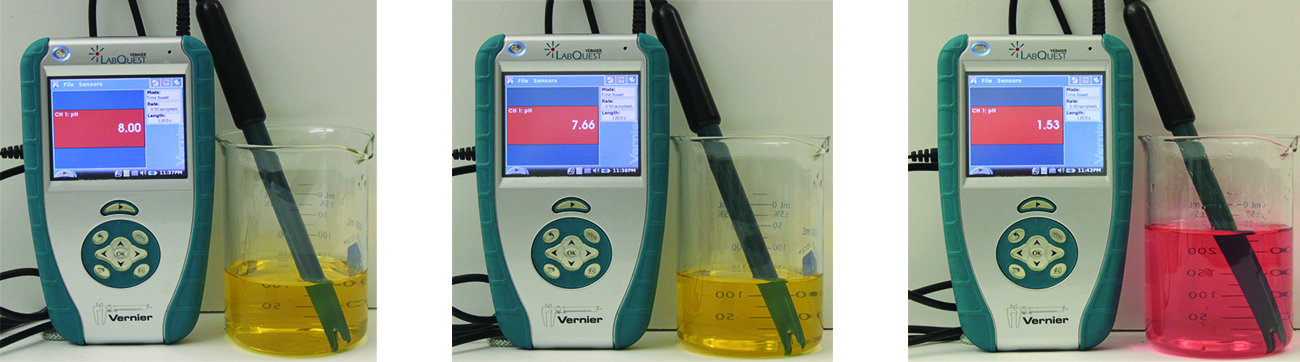Figure 8.6.3 – The indicator color (methyl orange) shows that a small amount of acid added to a buffered solution of pH 8 (beaker on the left) has little affect on the buffered system (middle beaker). However, a large amount of acid exhausts the buffering capacity of the solution and the pH changes dramatically (beaker on the right). (credit: modification of work by Mark Ott)

The buffer capacity is the amount of acid or base that can be added to a given volume of a buffer solution before the pH changes significantly, usually by one unit. Buffer capacity depends on the amounts of the weak acid and its conjugate base that are in a buffer mixture. For example, 1 L of a solution that is 1.0 M in acetic acid and 1.0 M in sodium acetate has a greater buffer capacity than 1 L of a solution that is 0.10 M in acetic acid and 0.10 M in sodium acetate even though both solutions have the same pH. The first solution has more buffer capacity because it contains more acetic acid and acetate ion.

## Selection of Suitable Buffer Mixtures

There are two useful rules of thumb for selecting buffer mixtures:

1. A good buffer mixture should have about equal concentrations of both of its components. A buffer solution has generally lost its usefulness when one component of the buffer pair is less than about 10% of the other. (Figure 8.6.4) shows how pH changes for an acetic acid-acetate ion buffer as base is added. The initial pH is 4.74. A change of 1 pH unit occurs when the acetic acid concentration is reduced to 11% of the acetate ion concentration.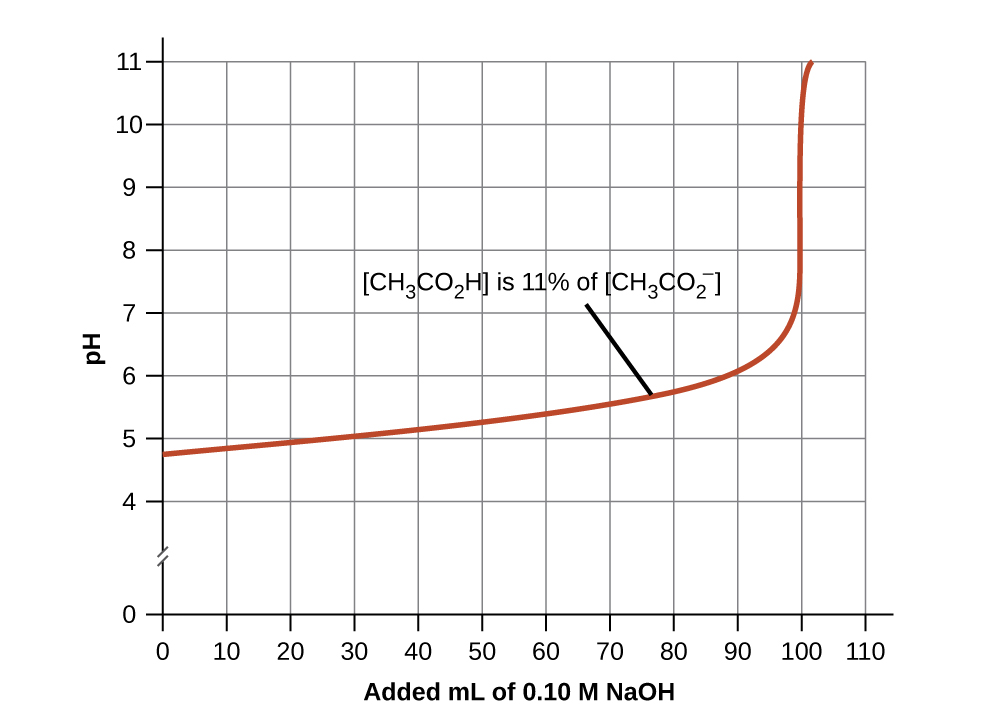Figure 8.6.4 – Change in pH as an increasing amount of a 0.10-M NaOH solution is added to 100 mL of a buffer solution in which, initially, [CH3CO2H] = 0.10 M and Note the greatly diminished buffering action occurring after the buffer capacity has been reached, resulting in drastic rises in pH on adding more strong base.
2. Weak acids and their salts are better as buffers for pHs less than 7; weak bases and their salts are better as buffers for pHs greater than 7.

Blood is an important example of a buffered solution, with the principal acid and ion responsible for the buffering action being carbonic acid, H2CO3, and the bicarbonate ion,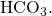When a hydronium ion is introduced to the blood stream, it is removed primarily by the reaction: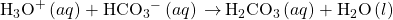An added hydroxide ion is removed by the reaction: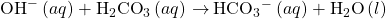The added strong acid or base is thus effectively converted to the much weaker acid or base of the buffer pair (H3O+ is converted to H2CO3 and OH is converted to HCO3). The pH of human blood thus remains very near the value determined by the buffer pairs pKa, in this case, 7.35. Normal variations in blood pH are usually less than 0.1, and pH changes of 0.4 or greater are likely to be fatal.

## The Henderson-Hasselbalch Equation

The ionization-constant expression for a solution of a weak acid can be written as: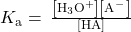Rearranging to solve for [H3O+] yields: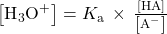Taking the negative logarithm of both sides of this equation gives: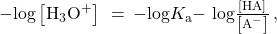which can be written as: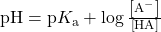where pKa is the negative of the logarithm of the ionization constant of the weak acid (pKa = −log Ka). This equation relates the pH, the ionization constant of a weak acid, and the concentrations of the weak conjugate acid-base pair in a buffered solution. Scientists often use this expression, called the Henderson-Hasselbalch equation, to calculate the pH of buffer solutions. It is important to note that the “x is small” assumption must be valid to use this equation.

Lawrence Joseph Henderson and Karl Albert Hasselbalch

Lawrence Joseph Henderson (1878–1942) was an American physician, biochemist and physiologist, to name only a few of his many pursuits. He obtained a medical degree from Harvard and then spent 2 years studying in Strasbourg, then a part of Germany, before returning to take a lecturer position at Harvard. He eventually became a professor at Harvard and worked there his entire life. He discovered that the acid-base balance in human blood is regulated by a buffer system formed by the dissolved carbon dioxide in blood. He wrote an equation in 1908 to describe the carbonic acid-carbonate buffer system in blood. Henderson was broadly knowledgeable; in addition to his important research on the physiology of blood, he also wrote on the adaptations of organisms and their fit with their environments, on sociology and on university education. He also founded the Fatigue Laboratory, at the Harvard Business School, which examined human physiology with specific focus on work in industry, exercise, and nutrition.

In 1916, Karl Albert Hasselbalch (1874–1962), a Danish physician and chemist, shared authorship in a paper with Christian Bohr in 1904 that described the Bohr effect, which showed that the ability of hemoglobin in the blood to bind with oxygen was inversely related to the acidity of the blood and the concentration of carbon dioxide. The pH scale was introduced in 1909 by another Dane, Sørensen, and in 1912, Hasselbalch published measurements of the pH of blood. In 1916, Hasselbalch expressed Henderson’s equation in logarithmic terms, consistent with the logarithmic scale of pH, and thus the Henderson-Hasselbalch equation was born.

Medicine: The Buffer System in Blood

The normal pH of human blood is about 7.4. The carbonate buffer system in the blood uses the following equilibrium reaction: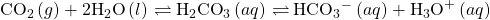The concentration of carbonic acid, H2CO3 is approximately 0.0012 M, and the concentration of the hydrogen carbonate ion,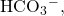is around 0.024 M. Using the Henderson-Hasselbalch equation and the pKa of carbonic acid at body temperature, we can calculate the pH of blood: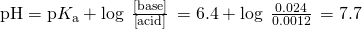The fact that the H2CO3 concentration is significantly lower than that of the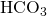ion may seem unusual, but this imbalance is due to the fact that most of the by-products of our metabolism that enter our bloodstream are acidic. Therefore, there must be a larger proportion of base than acid, so that the capacity of the buffer will not be exceeded.

Lactic acid is produced in our muscles when we exercise. As the lactic acid enters the bloodstream, it is neutralized by theion, producing H2CO3. An enzyme then accelerates the breakdown of the excess carbonic acid to carbon dioxide and water, which can be eliminated by breathing. In fact, in addition to the regulating effects of the carbonate buffering system on the pH of blood, the body uses breathing to regulate blood pH. If the pH of the blood decreases too far, an increase in breathing removes CO2 from the blood through the lungs driving the equilibrium reaction such that [H3O+] is lowered. If the blood is too alkaline, a lower breath rate increases CO2 concentration in the blood, driving the equilibrium reaction the other way, increasing [H+] and restoring an appropriate pH.

# Key Concepts and Summary

Solutions that contain appreciable amounts of a weak conjugate acid-base pair are called buffers. A buffered solution will experience only slight changes in pH when small amounts of acid or base are added. Addition of large amounts of acid or base can exceed the buffer capacity, consuming most of one conjugate partner and preventing further buffering action.

## Key Equations

• pKa = −log Ka
• pKb = −log Kb
•End of Chapter Exercises

(1) Explain why a buffer can be prepared from a mixture of NH4Cl and NaOH but not from NH3 and NaOH.

(2) Explain why the pH does not change significantly when a small amount of an acid or a base is added to a solution that contains equal amounts of the acid H3PO4 and a salt of its conjugate base NaH2PO4.

Solution

Excess H3O+ is removed primarily by the reaction: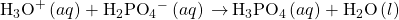Excess base is removed by the reaction: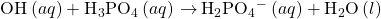(3) Explain why the pH does not change significantly when a small amount of an acid or a base is added to a solution that contains equal amounts of the base NH3 and a salt of its conjugate acid NH4Cl.

(4) What is [H3O+] in a solution of 0.25 M CH3CO2H and 0.030 M NaCH3CO2?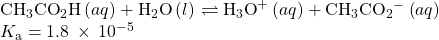Solution

[H3O+] = 1.510−4M

(5) What is [H3O+] in a solution of 0.075 M HNO2 and 0.030 M NaNO2?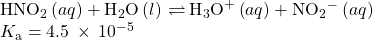(6) What is [OH] in a solution of 0.125 M CH3NH2 and 0.130 M CH3NH3Cl?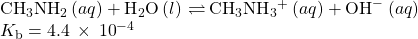Solution

[OH] = 4.210−4M

(7) What is [OH] in a solution of 1.25 M NH3 and 0.78 M NH4NO3?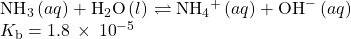(8) What is the effect on the concentration of acetic acid, hydronium ion, and acetate ion when the following are added to an acidic buffer solution of equal concentrations of acetic acid and sodium acetate:

(8a) HCl

(8b) KCH3CO2

(8c) NaCl

(8d) KOH

(8e) CH3CO2H

Solution

(a) The added HCl will increase the concentration of H3O+ slightly, which will react with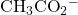and produce CH3CO2H in the process. Thus,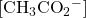decreases and [CH3CO2H] increases.

(b) The added KCH3CO2 will increase the concentration ofwhich will react with H3O+ and produce CH3CO2 H in the process. Thus, [H3O+] decreases slightly and [CH3CO2H] increases.

(c) The added NaCl will have no effect on the concentration of the ions.

(d) The added KOH will produce OH ions, which will react with the H3O+, thus reducing [H3O+]. Some additional CH3CO2H will dissociate, producingions in the process. Thus, [CH3CO2H] decreases slightly andincreases.

(e) The added CH3CO2H will increase its concentration, causing more of it to dissociate and producing moreand H3O+ in the process. Thus, [H3O+] increases slightly andincreases.

(9) What is the effect on the concentration of ammonia, hydroxide ion, and ammonium ion when the following are added to a basic buffer solution of equal concentrations of ammonia and ammonium nitrate:

(9a) KI

(9b) NH3

(9c) HI

(9d) NaOH

(9e) NH4Cl

(10) What will be the pH of a buffer solution prepared from 0.20 mol NH3, 0.40 mol NH4NO3, and just enough water to give 1.00 L of solution?

Solution

pH = 8.95

(11) Calculate the pH of a buffer solution prepared from 0.155 mol of phosphoric acid, 0.250 mole of KH2PO4, and enough water to make 0.500 L of solution.

(12) How much solid NaCH3CO2•3H2O must be added to 0.300 L of a 0.50-M acetic acid solution to give a buffer with a pH of 5.00? (Hint: Assume a negligible change in volume as the solid is added.)

Solution

37 g (0.27 mol)

(13) What mass of NH4Cl must be added to 0.750 L of a 0.100-M solution of NH3 to give a buffer solution with a pH of 9.26? (Hint: Assume a negligible change in volume as the solid is added.)

(14) A buffer solution is prepared from equal volumes of 0.200 M acetic acid and 0.600 M sodium acetate. Use 1.8010−5 as Ka for acetic acid.

(14a) What is the pH of the solution?

(14b) Is the solution acidic or basic?

(14c) What is the pH of a solution that results when 3.00 mL of 0.034 M HCl is added to 0.200 L of the original buffer?

Solution

(a) pH = 5.222; (b) The solution is acidic. (c) pH = 5.221

A 5.36 g sample of NH4Cl was added to 25.0 mL of 1.00 M NaOH and the resulting solution diluted to 0.100 L.

(15a) What is the pH of this buffer solution?

(15b) Is the solution acidic or basic?

(15c) What is the pH of a solution that results when 3.00 mL of 0.034 M HCl is added to the solution?

## Glossary

amount of an acid or base that can be added to a volume of a buffer solution before its pH changes significantly (usually by one pH unit)
mixture of appreciable amounts of a weak acid-base pair the pH of a buffer resists change when small amounts of acid or base are added
logarithmic version of the acid ionization constant expression, conveniently formatted for calculating the pH of buffer solutions